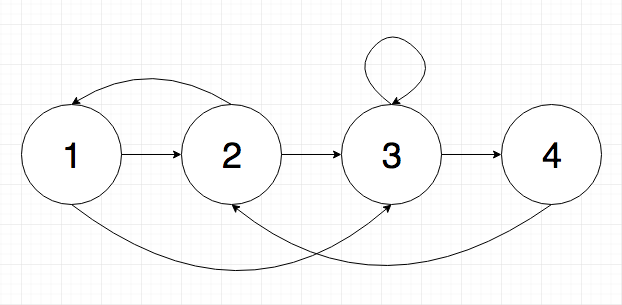# Coding Interview Question: Random Linked List## Question

Given a linked list where each node has two pointers, one to the next node and one to a random node in the list, clone the linked list.

eg.```1 -> 2 -> 3 -> 4 -> null
|    |    |    |
v    v    v    v
3    1    3    2
```

Once you think that you’ve solved the problem, click below to see the solution.

As always, remember that practicing coding interview questions is as much about how you practice as the question itself. Make sure that you give the question a solid go before skipping to the solution. Ideally if you have time, write out the solution first by hand and then only type it into your computer to verify your work once you've verified it manually. To learn more about how to practice, check out this blog post.

## Solution

How was that problem? You can check out the solution in the video below.

Here is the source code for the solution shown in the video (Github):

```// Private node class
private static class Node {
int value;
Node next;
Node random;
}

// Copy list using extra space. Store mapping of old nodes to new nodes
public static Node cloneExtraSpace(Node n) {
if (n == null) return n;

// Map nodes in old list to equivalent nodes in new list
HashMap mapping = new HashMap();

// Create new linked list, minus the random node pointers. Save mapping
// of equivalent old node to new node
Node copy = new Node();
Node nCurr = n, copyCurr = copy;
mapping.put(nCurr, copyCurr);

while (nCurr.next != null) {
copyCurr.next = new Node();
nCurr = nCurr.next;
copyCurr = copyCurr.next;
mapping.put(nCurr, copyCurr);
}

// Copy the random pointers. Find the random pointer in the original
// list and look up the equivalent using the map
nCurr = n;
copyCurr = copy;
while (nCurr != null) {
copyCurr.random = mapping.get(nCurr.random);
nCurr = nCurr.next;
copyCurr = copyCurr.next;
}

return copy;
}

// Copy list without using extra space. Interleave the nodes from the new
// with the nodes from the original list. Then separate the new list from
// the old
public static Node cloneNoExtraSpace(Node n) {
if (n == null) return n;

// Create new nodes in between the original nodes
Node nCurr = n;
while (nCurr != null) {
Node temp = new Node();
temp.value = nCurr.value;
temp.next = nCurr.next;
nCurr.next = temp;
nCurr = nCurr.next.next;
}

// Copy random pointers
nCurr = n;
while (nCurr != null) {
nCurr.next.random = nCurr.random.next;
nCurr = nCurr.next.next;
}

// Separate new nodes from old nodes
Node copy = n.next;
nCurr = n;
while (nCurr.next != null) {
Node tmp = nCurr.next;
nCurr.next = nCurr.next.next;
nCurr = tmp;
}

return copy;
}```